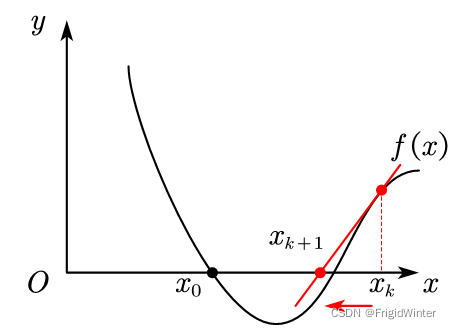﻿ 图文详解牛顿迭代算法原理及Python实现_python_脚本之家
python# 图文详解牛顿迭代算法原理及Python实现

## 1.引例## 2.牛顿迭代算法求根

f(x)=f(xk​)+f′(xk​)(x−xk​)+o(x)

xk+1​=xk​−f(xk​)/f'(xk​)​## 3.牛顿迭代优化

f(x)=f(xk​)+f′(xk​)(x−xk​)+1/2​f′′(xk​)(x−xk​)2+o(x)

f′(x)=f′(xk​)+f′′(xk​)(x−xk​)

xk+1​=xk​−f′(xk​)/f'′(xk​)​

xk+1​=xk​−[∇2f(xk​)]−1∇f(xk​)

① 以二阶速率向最优点收敛，迭代次数远小于梯度下降法，优化速度快；

②学习率为[∇2f(xk​)]−1 ，包含更多函数本身的信息，迭代步长可实现自动调整，可视为自适应梯度下降算法；

③ 耗费CPU计算资源多，每次迭代需要计算一次Hessian矩阵，且无法保证Hessian矩阵可逆且正定，因而无法保证一定向最优点收敛。

## 4 代码实战：Logistic回归

```import pandas as pd
import numpy as np
import os
import matplotlib.pyplot as plt
import matplotlib as mpl
from Logit import Logit

'''
* @breif: 从CSV中加载指定数据
* @param[in]: file -> 文件名
* @param[in]: colName -> 要加载的列名
* @param[in]: mode -> 加载模式, set: 列名与该列数据组成的字典, df: df类型
* @retval: mode模式下的返回值
'''
assert mode in ('set', 'df')
if mode == 'df':
return df
if mode == 'set':
res = {}
for col in colName:
res[col] = df[col].values
return res

if __name__ == '__main__':
# ============================
# 读取CSV数据
# ============================
csvPath = os.path.abspath(os.path.join(__file__, "../../data/dataset3.0alpha.csv"))
dataX = loadCsvData(csvPath, ["含糖率", "密度"], 'df')
label = np.array([
1 if i == "是" else 0
for i in list(map(lambda s: s.strip(), list(dataY['好瓜'])))
])

# ============================
# 绘制样本点
# ============================
line_x = np.array([np.min(dataX['密度']), np.max(dataX['密度'])])
mpl.rcParams['font.sans-serif'] = [u'SimHei']
plt.title('对数几率回归模拟\nLogistic Regression Simulation')
plt.xlabel('density')
plt.ylabel('sugarRate')
plt.scatter(dataX['密度'][label==0],
dataX['含糖率'][label==0],
marker='^',
color='k',
s=100,
label='坏瓜')
plt.scatter(dataX['密度'][label==1],
dataX['含糖率'][label==1],
marker='^',
color='r',
s=100,
label='好瓜')

# ============================
# 实例化对数几率回归模型
# ============================
logit = Logit(dataX, label)

# 采用牛顿迭代法
logit.logitRegression(logit.newtomMethod)
line_y = -logit.w[0, 0] / logit.w[1, 0] * line_x - logit.w[2, 0] / logit.w[1, 0]
plt.plot(line_x, line_y, 'g-', label="牛顿迭代法")

# 绘图
plt.legend(loc='upper left')
plt.show()
```

```    '''
* @breif: 牛顿迭代法更新权重
* @param[in]: None
* @retval: 优化参数的增量dw
'''
def newtomMethod(self):
wTx = np.dot(self.w.T, self.X).reshape(-1, 1)
p = Logit.sigmod(wTx)
dw_1 = -self.X.dot(self.y - p)
dw_2 = self.X.dot(np.diag((p * (1 - p)).reshape(self.N))).dot(self.X.T)
dw = np.linalg.inv(dw_2).dot(dw_1)
return dw

```